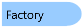﻿ Factory Class
Factory Class
Members  Example

The Factory class provides static methods for creating instances of IWorkbookSet and IWorkbook.
Object ModelSyntax
```'Declaration

Public MustInherit Class Factory ```
```'Usage

Dim instance As Factory```
`public abstract class Factory `
Remarks
All methods in Factory are thread safe.
Example
```class Program
{
static void Main(string[] args)
{
// Create a new empty workbook set.
// Create a new empty workbook in the workbook set.
// Get a reference to the first worksheet.
// Get a reference to the top left cell of Sheet1.
// Set a formula.
a1.Formula = "=24901.55 / PI()";
// Output the result of the formula.
System.Console.WriteLine("The diameter of the earth is " + a1.Value.ToString() + " miles.");
}
}```
```Module Program
Sub Main()
' Create a new empty workbook set.
' Create a new empty workbook in the workbook set.
' Get a reference to the first worksheet.
Dim worksheet As SpreadsheetGear.IWorksheet = workbook.Worksheets("Sheet1")
' Get a reference to the top left cell of Sheet1.
Dim a1 As SpreadsheetGear.IRange = worksheet.Cells("A1")
' Set a formula.
a1.Formula = "=24901.55 / PI()"
' Output the result of the formula.
Console.WriteLine(("The diameter of the earth is " + a1.Value.ToString() + " miles."))
End Sub
End Module```
Inheritance Hierarchy

System.Object